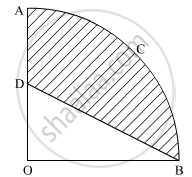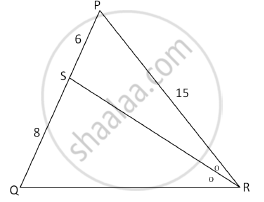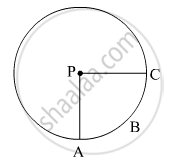# ICSE Class 10 - CISCE Important Questions for Mathematics

Subjects
Topics
Subjects
Popular subjects
Topics
Mathematics
< prev  1 to 20 of 379  next >

In the given figure, OACB is a quadrant of a circle with centre O and radius 3.5 cm. If OD = 2 cm, find the area of the shaded region.Appears in 4 question papers
Chapter: [0.02] Circles
Concept: Areas of Sector and Segment of a Circle

If the quadratic equation px2 − 2√5px + 15 = 0 has two equal roots then find the value of p.

Appears in 3 question papers
Concept: Nature of Roots

In the following figure, in ΔPQR, seg RS is the bisector of ∠PRQ. If PS = 6, SQ = 8, PR = 15, find QR.Appears in 3 question papers
Chapter: [0.05] Similarity
Concept: Similarity of Triangles

In the given figure, if A(P-ABC) = 154 cm2  radius of the circle is 14 cm, find

(1) ∠APC

(2) l ( arc ABC) .Appears in 2 question papers
Chapter: [0.03] Constructions
Concept: Circumference of a Circle

In the given figure, if A(P-ABC) = 154 cm2  radius of the circle is 14 cm, find

(1) ∠APC

(2) l ( arc ABC) .Appears in 2 question papers
Chapter: [0.04] Mensuration
Concept: Circumference of a Circle

Jaya borrowed Rs. 50,000 for 2 years. The rates of interest for two successive years are 12% and 15% respectively. She repays 33,000 at the end of the first year. Find the amount she must pay at the end of the second year to clear her debt.

Appears in 1 question paper
Chapter: [0.01] Compound Interest
Concept: Concept of Compound Interest > Compound Interest as a Repeated Simple Interest Computation with a Growing Principal

P(1, -2) is a point on the line segment A(3, -6) and B(x, y) such that AP : PB is equal to 2 : 3. Find the coordinates of B.

Appears in 1 question paper
Chapter: [0.01] Co-ordinate Geometry Distance and Section Formula
Concept: Co-ordinates Expressed as (x,y)

Mr Lalit invested Rs. 5000 at a certain rate of interest, compounded annually for two years. At the end of the first year, it amounts to Rs. 5325. Calculate

1) The rate of interest

2) The amount at the end of the second year, to the nearest rupee.

Appears in 1 question paper
Chapter: [0.01] Compound Interest
Concept: Concept of Compound Interest > Use of Compound Interest in Computing Amount Over a Period of 2 Or 3-years

In what time will Rs. 1500 yield Rs. 496.50 as compound interest at 10% per annum compounded annually?

Appears in 1 question paper
Chapter: [0.01] Compound Interest
Concept: Concept of Compound Interest > Use of Compound Interest in Computing Amount Over a Period of 2 Or 3-years

Use ruler and compasses only for the following questions. All constructions lines and arcs must be clearly shown.

Construct the locus of points at a distance of 3.5 cm from A.

Appears in 1 question paper
Chapter: [0.01] Loci
Concept: Loci Examples

Use ruler and compasses only for the following questions. All constructions lines and arcs must be clearly shown

Construct the locus of points equidistant from AC and BC.

Appears in 1 question paper
Chapter: [0.01] Loci
Concept: Loci Examples

Use ruler and compasses only for the following questions. All constructions lines and arcs must be clearly shown

Mark 2 points X and Y which are a distance of 3.5 cm from A and also equidistant from AC and BC. Measure XY.

Appears in 1 question paper
Chapter: [0.01] Loci
Concept: Loci Examples

The present population of the town is 2,00,000. The population is increased by 10% in the first year and 15% in the second year. Find the population of the town at the end of two years.

Appears in 1 question paper
Chapter: [0.01] Compound Interest
Concept: Concept of Compound Interest > Use of Compound Interest in Computing Amount Over a Period of 2 Or 3-years

If a, b, c are in continued proportion, prove that (a + b + c) (a – b + c) = a2 + b2 + c2

Appears in 1 question paper
Chapter: [0.01] Compound Interest
Concept: Concept of Compound Interest > Compound Interest as a Repeated Simple Interest Computation with a Growing Principal

Construct a triangle ABC with AB = 5.5 cm, AC = 6 cm and ∠BAC = 105°

Hence:

1) Construct the locus of points equidistant from BA and BC

2) Construct the locus of points equidistant from B and C.

3) Mark the point which satisfies the above two loci as P. Measure and write the length of PC.

Appears in 1 question paper
Chapter: [0.01] Loci
Concept: Constructions Under Loci

Ranbir borrows Rs. 20,000 at 12% per annum compound interest. If he repays Rs. 8400 at the end of the first year and Rs. 9680 at the end of the second year, find the amount of loan outstanding at the beginning of the third year.

Appears in 1 question paper
Chapter: [0.01] Compound Interest
Concept: Concept of Compound Interest > Use of Compound Interest in Computing Amount Over a Period of 2 Or 3-years

The value of 'a' for which of the following points A(a, 3), B (2, 1) and C(5, a) a collinear. Hence find the equation of the line.

Appears in 1 question paper
Chapter: [0.01] Co-ordinate Geometry Distance and Section Formula
Concept: Distance Formula

The compound interest, calculated yearly, on a certain sum of money for the second year is Rs. 1320 and for the third year is Rs. 1452. Calculate the rate of interest and the original sum of money

Appears in 1 question paper
Chapter: [0.01] Compound Interest
Concept: Concept of Compound Interest > Use of Compound Interest in Computing Amount Over a Period of 2 Or 3-years

At what rate % p.a. will a sum of Rs. 4000 yield Rs. 1324 as compound interest in 3 years?

Appears in 1 question paper
Chapter: [0.01] Compound Interest
Concept: Concept of Compound Interest > Compound Interest as a Repeated Simple Interest Computation with a Growing Principal

On what sum of money will the difference between the compound interest and simple interest for 2 years be equal to Rs. 25 if the rate of interest charged for both is 5% p.a.?

Appears in 1 question paper
Chapter: [0.01] Compound Interest
Concept: Concept of Compound Interest > Compound Interest as a Repeated Simple Interest Computation with a Growing Principal
< prev  1 to 20 of 379  next >